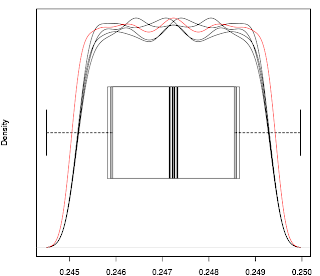## Tuesday, 27 April 2010

### Monte Carlo Optimisation of Control Limits #2Having run the routine again on sine waves of various amplitudes I have plotted boxplots and kernal density plots of the ucl and lcl optimised multipliers for sine waves of periods n=10 to 50 inclusive, using R. Visual inspection of the plots suggest that, in general* (see note below), there are no real differences between the distributions and a typical plot is shown above (again this is for a sine wave of period n=20). The black plots are
• optimised multiplier for ucl of sine wave with amplitude of 1
• optimised multiplier for lcl of sine wave with amplitude of 1
• optimised multiplier for ucl of sine wave with variable amplitude
• optimised multiplier for lcl of sine wave with variable amplitude
The red line is a kernal density plot of all four of the above combined into a single, global distribution for that period sine wave.

Obviously just looking at plots is not statistically robust, so the next thing I did was to run Kolmogorov-Smirnov tests in R to see if the null hypothesis that the four separate distributions come from the same distribution can be rejected. For this purpose I used a p-value of 0.05 for the significance level. The comparative test runs were
1. ucl sine wave amplitude 1 and lcl sine wave amplitude 1
2. ucl sine wave variable amplitude and lcl sine wave variable amplitude
3. ucl sine wave amplitude 1 and ucl sine wave variable amplitude
4. lcl sine wave amplitude 1 and lcl sine wave variable amplitude
The logic behind this choice is:-
if A=B and C=D and A=C and B=D then A=B=C=D.

The actual test in R that was used was the Bootstrap Kolmogorov-Smirnov package ks.boot(), the R documentation description for which is quoted below:-

"This function executes a bootstrap version of the univariate Kolmogorov-Smirnov test which provides correct coverage even when the distributions being compared are not entirely continuous. Ties are allowed with this test unlike the traditional Kolmogorov-Smirnov test"

It is obvious from the discrete nature of the raw data being tested that this test is more appropriate than the traditional Kolmogorov-Smirnov test, but more importantly the symmetrical nature of a sine wave almost guarantees that there are likely to be ties in the data, hence the choice of this test. The number of bootstraps performed for each test was n=1000. The p-value was sufficiently low to reject the null hypothesis for the following periods (numbered bullet points correspond to tests identified above)

1. Periods 16 ,24, 32, 40 and 48
2. Periods 16, 24, 27, 32, 40, 45, 48, 49
3. Periods 38 and 49
4. Periods 38 and 49

*The exceptions to the general pattern mentioned above will be the subject of a later blog entry.